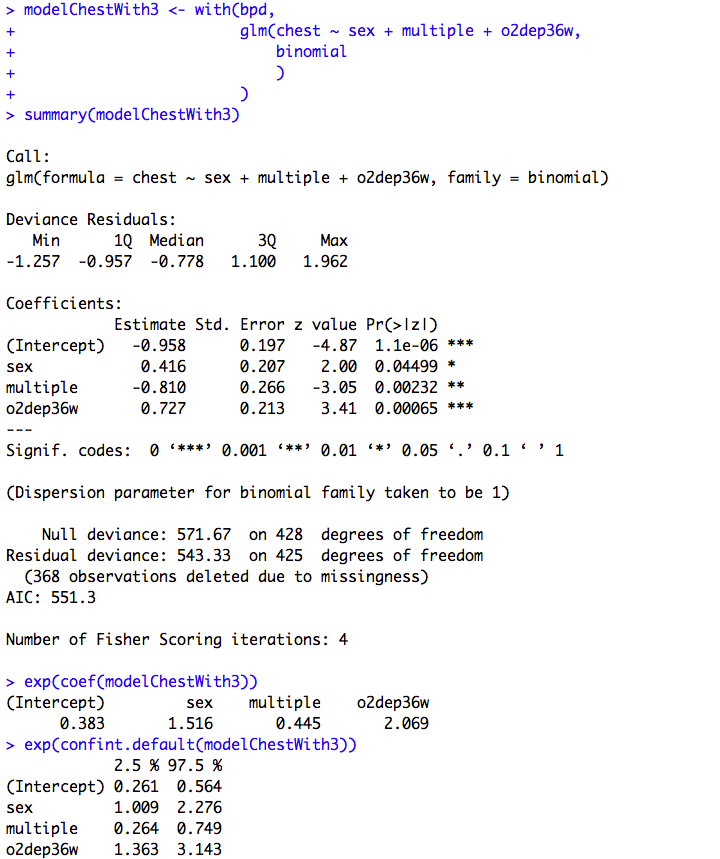﻿ Chapter 10: Multifactorial analyses using R

# Chapter 10: Multifactorial analyses using R

### Box 10.3 Presenting the results for one-way analysis of variance### Box 10.5 Presenting the results of multiple regression### Box 10.8 Presentation of multiple regression with categorical predictor variable### Box 10.12 Presenting the results for logistic regression### Box 10.14 Presenting logistic regression with categorical predictor variables### Figure 10.6 and Box 10.14 Code